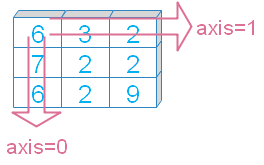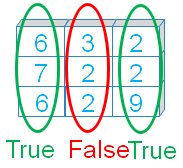# Numpy max()

Numpy

``numpy.max(a,axis=None,out=None,keepdims, initial, where)``
Return highest of elements across given axis.
 `a` array, elements to get the max value `axis` Int (optional ), or tuple, default is None, maximum value among all the elements. If axis given then values across the axis is returned. `out` Optional. If given then output to be stored. Must be of same shape as of the output `keepdims` Bool ( Optional ), output matches to the input array dimension. `where` Optional, Elements to include for calculation of maximum value `initial` Optional, int, Minimum value of the output. If given, then this is considered if it is more than the actual output
We will use these parameters in our examples.

## Sample array

You can use randint() to create an array for our examples. Or can use fixed elements to create the array.
``````import numpy as np
# my_data=np.random.randint(2,high=7,size=(3,3),dtype='int16')
my_data=np.array([[6, 3, 2], [7, 2, 2], [6, 2, 9]])
print(my_data)``````
Output
``````[[6 3 2]
[7 2 2]
[6 2 9]]``````

## Axis

Maximum vlaue of the elements across the axis.``````print("max()      : ", my_data.max())
print("max(axis=0): ", my_data.max(axis=0))
print("max(axis=1): ", my_data.max(axis=1))``````
Output
``````max()       :  9
max(axis=0) :  [7 3 9]
max(axis=1) :  [6 7 9]``````

## out

Alternative output array, must be of same shape as expected output. Let us first check with axis.
``````x = np.zeros(3,dtype=int)
print(my_data.max(axis=0,out=x))
print(x)``````
Output
``````[7 3 9]
[7 3 9]``````
Without using axis
``````y = np.array(1)
print(my_data.max(out=y))
print(y)``````
Output
``````9
9``````

## keepdims

If it is set to True ( keepdims=True ) then it will take the dimension of input array.
``````print("max(keepdims=True) : ", my_data.max(keepdims=True))
print("max(keepdims=False) : ", my_data.max(keepdims=False))``````
Output
``````max(keepdims=True) :  []
max(keepdims=False) :  9``````

## Using whereBy using where we can say which elements to use and which elements not to use ( by setting True or False ) . While using where we have to give initial value.
``print(my_data.max(where=[True, False,True],initial=2))``
Output
``6``
Using axis with where
``````print(my_data.max(axis=1,where=[True, False,True],initial=2))
print(my_data.max(axis=0,where=[True, False,True],initial=2))``````
Output
``````[6 2 6]
[6 2 3]``````

## initial

We can assign initial value to our output. Note that the final output will be maximum of initial vlaue and actual min value ( without the initial value ).
``````print(my_data.max())  # 9
print(my_data.max(initial=2)) # 9
print(my_data.max(initial=12)) # 12 ``````
Output
``````9
9
12``````
Check the where option above. The value assigned to intial value is given as output where it is less than the actual output.

## Intial vlaue with Axis option

Compare the values with initial value and without intitial value. We used axis=0 , you can try with axis=1.
``````print(my_data.max(axis=0))  # [7 3 9]
print(my_data.max(axis=0,initial=2)) # [7 3 9]
print(my_data.max(axis=0,initial=12)) # [12 12 12]``````
Output
``````[7 3 9]
[7 3 9]
[12 12 12]``````

Subscribe to our YouTube Channel here

## Subscribe

* indicates required
Subscribe to plus2netplus2net.com